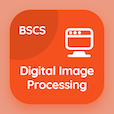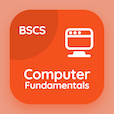Computer Science Online Courses

Digital Image Processing Quizzes

Digital Image Processing Quiz PDF - Complete

# Fundamentals of Image Compression Quiz MCQ Online p. 45

Practice Fundamentals of Image Compression quiz questions and answers PDF, fundamentals of image compression trivia questions 45 to learn online Digital Image Processing course for online classes. Image Compression MCQ questions, fundamentals of image compression Multiple Choice Questions (MCQ) for online college degrees. "Fundamentals of Image Compression Quiz" PDF eBook: extension to functions of two variables, histogram matching, dip: color fundamentals, point line and edge detection in image processing, fundamentals of image compression test prep for computer science associate degree.

"System of symbols to represent event is called" MCQ PDF: word, storage, code, and nibble for computer software engineer online degree. Solve image compression questions and answers to improve problem solving skills for 2 year computer science degree.

## Trivia Quiz on Fundamentals of Image Compression MCQs

MCQ: System of symbols to represent event is called

storage
word
code
nibble

MCQ: For point detection we use

first derivative
second derivative
third derivative
Both A and B

MCQ: Three primary colors are

Red, green, blue
Red, cyan, blue
Red, white, black
Red, green, yellow

MCQ: Method generating a processed image having specified histogram is called

histogram enhancement
histogram normalization
histogram equalization
histogram matching

MCQ: A continuous band limited function can be recovered with no error if sampled intervals are less than 1/2umax is the statement of

2D sampling series
3D sampling theorem
1D sampling theorem
2D sampling theorem

### More Quizzes from Digital Image Processing Course